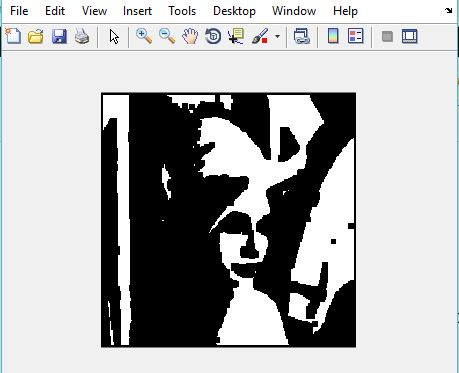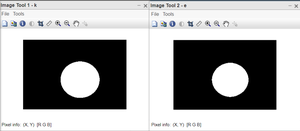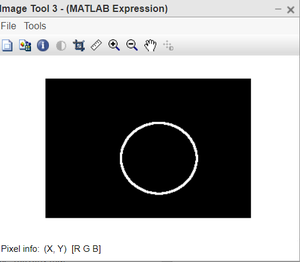# Matlab | Erosion of an Image

Morphology is known as the broad set of image processing operations that process images based on shapes. It is also known as a tool used for extracting image components that are useful in the representation and description of region shape.

The basic morphological operations are:

• Erosion
• Dilation

Erosion:

• Erosion shrink-ens the image pixels i.e. it is used for shrinking of element A by using element B.
• Erosion removes pixels on object boundaries.:
• The value of the output pixel is the minimum value of all the pixels in the neighborhood. A pixel is set to 0 if any of the neighboring pixels have the value 0.

Approach:

• Using function im2bw(), convert the RGB image to a binary image.
• Create a structuring element or you can use any predefined mask eg. special(‘sobel’).
• Store the number of rows and columns in an array and loop through it.
• Create a zero matrix of the size same as the size of our image.
• Leaving the boundary pixels start moving the structuring element on the image and start comparing the pixel with the pixels present in the neighborhood.
• If the value of the neighborhood pixel is 0, then change the value of that pixel to 0.

Example:

## MATLAB

 `% Matlab code for Erosion` `% read image` `I=imread(``'lenna.png'``);   `   `% convert to binary  ` `I=im2bw(I); `   `% create structuring element              ` `se=ones(5, 5);`   `% store number of rows ` `% in P and number of columns in Q.            ` `[P, Q]=size(se); `   `% create a zero matrix of size I.        ` `In=zeros(size(I, 1), size(I, 2)); `   `for` `i=ceil(P/2):size(I, 1)-floor(P/2)` `    ``for` `j=ceil(Q/2):size(I, 2)-floor(Q/2)`   `        ``% take all the neighbourhoods.` `        ``on=I(i-floor(P/2):i+floor(P/2), j-floor(Q/2):j+floor(Q/2)); `   `        ``% take logical se` `        ``nh=on(logical(se)); ` `      `  `        ``% compare and take minimum value of the neighbor ` `        ``% and set the pixel value to that minimum value. ` `        ``In(i, j)=min(nh(:));      ` `    ``end` `end`   `imshow(In);`

Output:figure: Input imagefigure: Output Image

Let’s take another image to perform Erosion and here we use different MATLAB functions.

Syntax:

• strel() function is used to define the structuring element. We have chosen disk-shaped SE, of radius 5.
• imerode() function is used to perform the erosion operation.
• imtool() function is used to display the image.

Example:

## Matlab

 `% MATLAB code for Erison` `% read the image.` `k=imread(``"erosion.png"``);`   `%define the structuring element.` `SE=strel(``'disk'``,5);`   `%apply the erosion operation.` `e=imerode(k,SE);`   `%display all the images.` `imtool(k,[]);` `imtool(e,[]);`   `%see the effective reduction in org,image` `imtool(k-e,[]);`

Output:Figure: Left: Original image, Right: Eroded imageFigure: Output image

Code explanation:

• SE=strel(‘disk’,5); this line defines the structuring element.
• e=imerode(k,SE); this line applies the erosion operation.
• imtool(k,[]); this line displays the original image.
• imtool(e,[]); this line displays the eroded image.
• imtool(k-e,[]); this line shows the effective reduction in original image.

The last image shows the extent to which the original image got eroded. We have used the Structuring element of disk-shaped and the image we used is also circular in shape. This gives us the very desired output to understand erosion.

Whether you're preparing for your first job interview or aiming to upskill in this ever-evolving tech landscape, GeeksforGeeks Courses are your key to success. We provide top-quality content at affordable prices, all geared towards accelerating your growth in a time-bound manner. Join the millions we've already empowered, and we're here to do the same for you. Don't miss out - check it out now!

Previous
Next